• 机器学习的讲义，主要介绍利用矩阵和最大似然估计推导最小均方误差函数
• 本文将从代价函数的个人理解，均方误差代数函数作用原理以及在线性回归问题中为什么选择均方误差代数函数阐述。 1、代价函数的理解： 代价函数：真实的值与预测的值之间的偏差，由于偏差有可能正有可能负，因此使用...
在机器学习中，线性回归、逻辑回归问题总是绕不开代价函数。本文将从代价函数的个人理解，均方误差代数函数作用原理以及在线性回归问题中为什么选择均方误差代数函数阐述。
1、代价函数的理解：
代价函数：真实的值与预测的值之间的偏差，由于偏差有可能正有可能负，因此使用均方差来表示。代价函数的目的即用于找到最优解。
损失函数Loss Function：定义在单个样本上，算的是一个样本的误差。 代价函数Cost Function：定义在整个训练集上，算的是所有样本的误差，也就是损失函数的平均。 目标函数Object Function：定义为最终需要优化的函数。 Object Function = Cost Function（经验风险） + 正则化项（结构风险）
2、均方误差代数函数作用原理：
对于回归问题，代价函数常选择均方误差函数。下面阐述其作用原理： 对于假设函数：

h

θ

(

x

)

=

θ

0

+

θ

1

x

h_{\theta}(x)=\theta_{0}+\theta_{1} x

参数

θ

0

\theta_{0}

和

θ

1

\theta_{1}

两个参数的变化会导致假设函数的变化: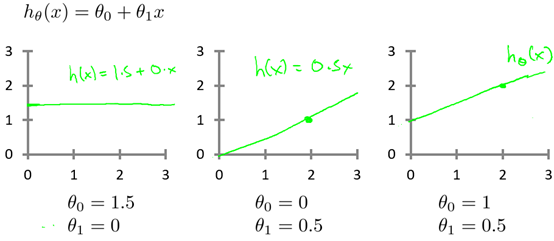实际的例子中，数据会以很多点的形式给出，回归问题就是将这些点拟合成一条曲线，即找到最优的参数

θ

0

\theta_{0}

和

θ

1

\theta_{1}

使得拟合的曲线更能代表所有的数据。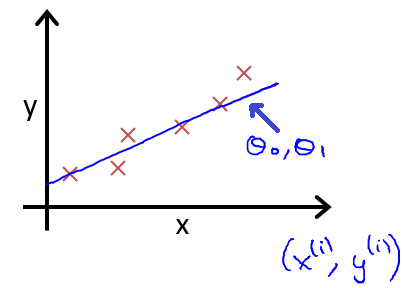如何找到最优参数

θ

0

\theta_{0}

和

θ

1

\theta_{1}

呢？使用代价函数！以均方误差代数函数为例： 从最简单的单一参数来看，假设函数为：

h

θ

(

x

)

=

θ

1

x

h_{\theta}(x)=\theta_{1} x

均方误差代数函数的主要思想就是将实际数据给出的值与拟合出的线的对应的值做差，求出拟合出的直线与实际的差距。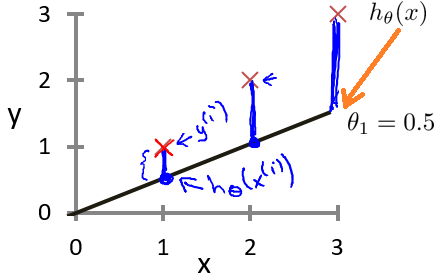为了使这个值不受个别极端数据的影响而产生巨大波动，采用类似方差再取二分之一的方式来减小个别数据影响。 这样，就产生了代价函数：

J

(

θ

0

,

θ

1

)

=

1

2

m

∑

i

=

1

m

(

h

θ

(

x

(

i

)

)

−

y

(

i

)

)

2

J\left(\theta_{0},\theta_{1}\right)=\frac{1}{2m}\sum_{i=1}^{m}\left(h_{\theta}\left(x^{(i)}\right)-y^{(i)}\right)^{2}

而最优解即为代价函数的最小值，根据以上公式多次计算可得到代价函数的图像：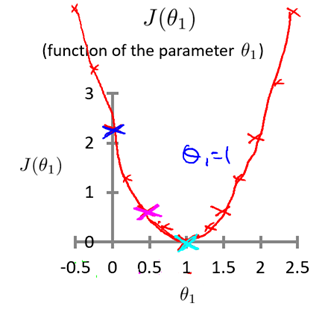可以轻易知道该代价函数的最小值(如何求解最小值？梯度下降算法~)，这时可以解出对应的 如果更多参数的话，图像就会更为复杂，两个参数的时候就已经是三维图像：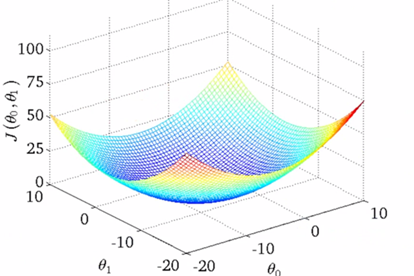高度即为均方误差代价函数的值，若参数再多则无法进行可视化，但其原理是相似的。 因此，我们可以终结得到：对于回归问题的代价函数，其最小值为：

minimize

⁡

θ

0

,

θ

1

J

(

θ

0

,

θ

1

)

\underset{\theta_{0}, \theta_{1}}{\operatorname{minimize}} J\left(\theta_{0}, \theta_{1}\right)

3、在回归问题中为什么选择均方误差函数：
从代价函数的理解考虑：代价是预测值和实际值之间的差距（两点之差），对于多个样本来说，就是差距之和。 将每一个样本的预测值和实际值之差相加，这不就是代价？但是注意到就每一个样本而言，代价有正有负，所有样本的代价加起来有可能出现正负抵消的情况，所以这并不是一个合适的代价函数（指将每一个样本的预测值和实际值之差相加）。 解决有正负的问题： 使用绝对值函数来表示代价，为了方便计算最小代价（计算最小代价可能用到最小二乘法），直接使用平方来衡量代价，即使用绝对值的平方来表示单个样本的代价，那么一个数据集的代价为：

(

h

θ

(

x

(

i

)

)

−

y

(

i

)

)

2

\left(h_{\theta}\left(x^{(i)}\right)-y^{(i)}\right)^{2}

即代价函数为：

J

(

θ

0

,

θ

1

)

=

1

2

m

∑

i

=

1

m

(

h

θ

(

x

(

i

)

)

−

y

(

i

)

)

2

J\left(\theta_{0},\theta_{1}\right)=\frac{1}{2m}\sum_{i=1}^{m}\left(h_{\theta}\left(x^{(i)}\right)-y^{(i)}\right)^{2}

为什么分母取2

m

m

而非

m

m

，是为了计算方便。
展开全文代价函数 线性回归
• 计算均方误差MSE信噪比SNR峰值信噪比PSNR绝对平均误差的matlab函数
• 有助于目录的生成如何改变文本的样式插入链接与图片如何插入一段漂亮的代码片生成一个适合你的列表创建一个表格设定内容居中、居左、居右...列表如何创建一个注脚注释也是必不可少的KaTeX数学公式新的甘特图功能...
均方误差代价函数 MSE Cost Function
代价函数可以用来衡量假设函数(hypothesis function)的准确性
在线性回归中，如果假设函数为h(x)=θ0+θ1*x, 均方误差代价函数公式如下图: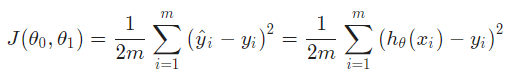θ0 和 θ1是假设函数的参数 h(x)就是预测值 m是训练的样本数量 i表示第i个样本
均方误差函数就是把预测值和真实值差的平方求和再除以2倍的样本数量
其中取2m而不取m是为了方便计算梯度.
用一道题帮助我们理解代价函数: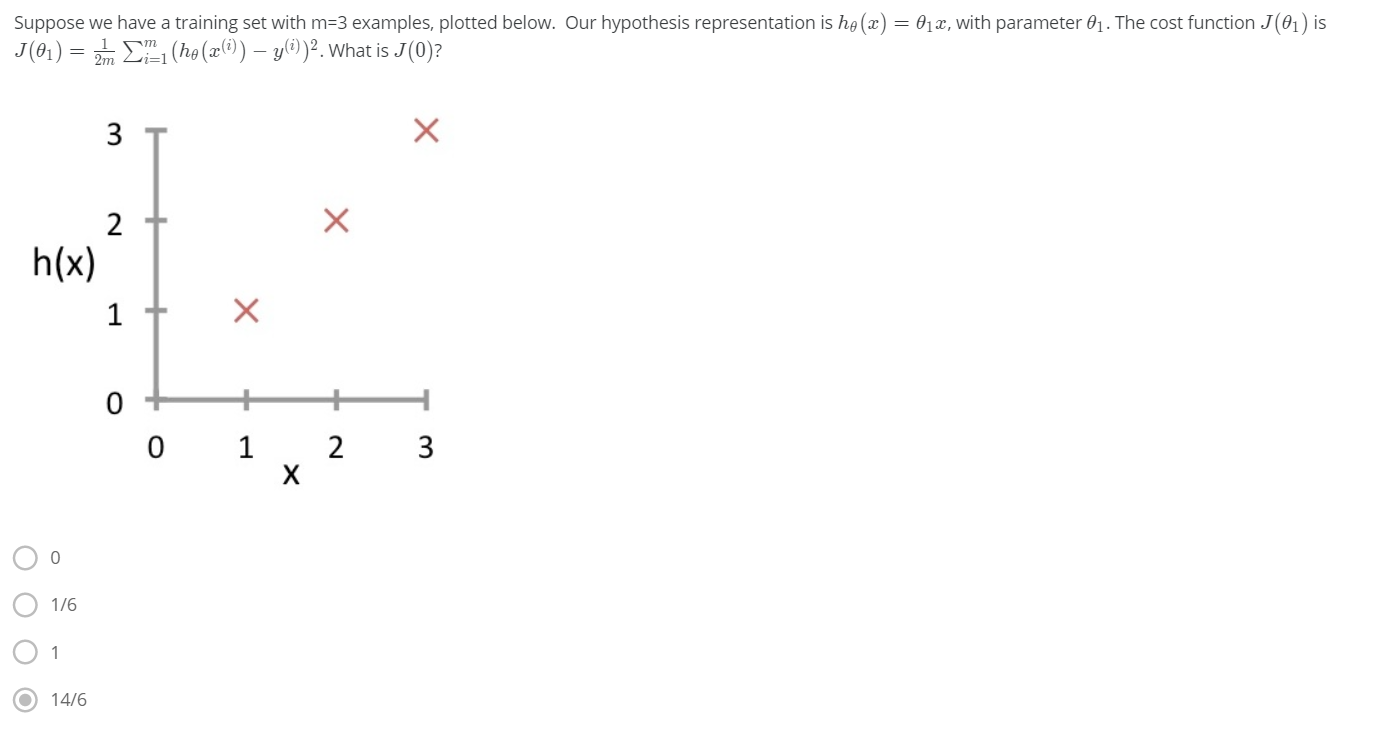这道题给了三个样本点，并且给出假设函数h(x)=θ1x,代价函数是均方误差代价函数，求J(0) 由题可知，θ0=0，要求J(0)，就是让θ1=0，当θ1=0的时候，其实h(x)=0 那么根据公式可以计算，选D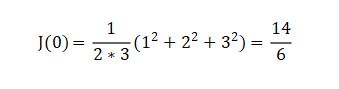当代价函数J(θ0，θ1)最小的时候，假设函数的预测值和真实值最接近 如下图，给定三个样本点(1,1),(2,2),(3,3),对于h(x)=0+θ1x,当θ1=1时，J(θ0,θ1)=0,即预测值和真实值完全相同，当θ1不为1时，预测的偏差随着θ1距离1的远近程度增加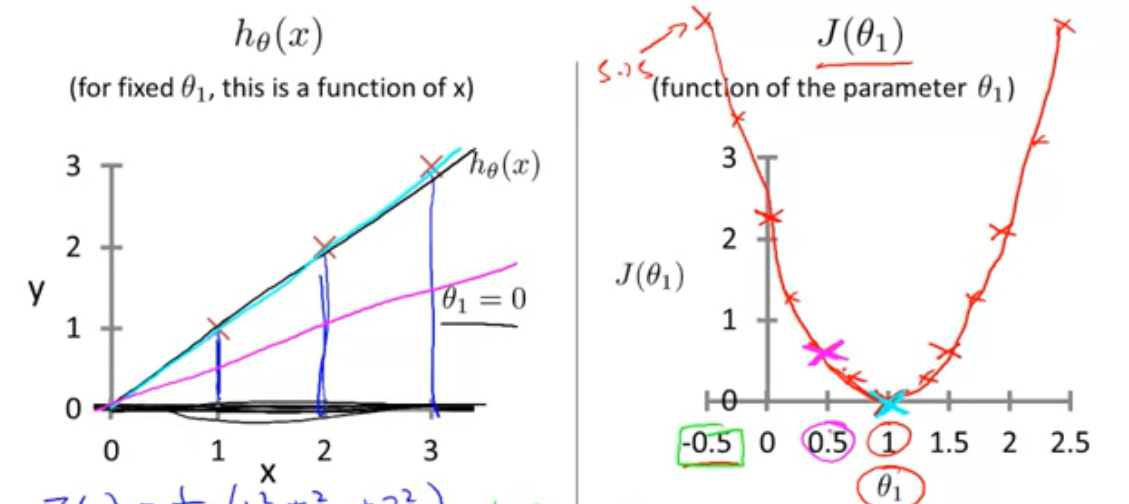展开全文机器学习
• 使用matlab对图象计算均方误差使用matlab对图象计算均方误差matlab
• ** 线性回归均方损失函数公式推导过程 ** 参考：https://www.cnblogs.com/ZhongliangXiang/p/8016635.html
**
线性回归均方损失函数公式推导过程
** 参考：https://www.cnblogs.com/ZhongliangXiang/p/8016635.html
展开全文神经网络
• 损失函数（loss function）可以为任意函数，常用的是均方误差和交叉熵误差 损失函数：最优参数解的参考指标，希望它越小越好 用于评价神经网络的性能“恶劣程度”，当前神经网络对监督数据（训练数据）在多大程度上...
损失函数（loss function）可以为任意函数，常用的是均方误差和交叉熵误差损失函数：最优参数解的参考指标，希望它越小越好用于评价神经网络的性能“恶劣程度”，当前神经网络对监督数据（训练数据）在多大程度上不拟合，不一致
均方误差（mean squared error）：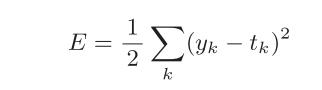yk：神经网络的输出 tk：监督数据（训练数据）
mean_squared_error.py
# oding: utf-8

# 损失函数之均方误差
import numpy as np

def mean_squared_error(y, t):
return 0.5 * np.sum((y - t) ** 2)

# 手写数字识别MNIST
t = [0, 0, 1, 0, 0, 0, 0, 0, 0, 0] # 设置“2”为正确解，监督数据
y = [0.1, 0.05, 0.6, 0.0, 0.05, 0.1, 0.0, 0.1, 0.0, 0.0] # softmax输出激活函数故可以理解为概率，设置‘2’为最高概率
print(mean_squared_error(np.array(y), np.array(t))) # 0.09750000000000003 损失函数值很低

y = [0.1, 0.05, 0.1, 0.0, 0.05, 0.1, 0.0, 0.6, 0.0, 0.0] # 设置‘7’为最高概率的情况为（0.6）
print(mean_squared_error(np.array(y), np.array(t))) # 0.5975 损失函数值很高
展开全文python
• 均方误差损失函数（MSE，mean squared error 回归问题解决的是对具体数值的预测，比如房价预测、销量预测等等，解决回归问题的神经网络一般只有一个输出节点，这个节点的输出值就是预测值。本文主要介绍回归问题下的...
• 比如对于一个4分类问题，期望输出应该为g0=[0,1,0,0]，实际输出为g1=[0.2,0.4,0.4,0]，计算g1与g0之间的差异所使用的方法，就是损失函数，分类问题中常用损失函数是交叉熵。 交叉熵（cross entropy）描述的是两个...
• MSE均方误差（L2 loss） 1.代码展示MAE和MSE图片特性 import tensorflow as tf import matplotlib.pyplot as plt sess = tf.Session() x_val = tf.linspace(-1.,-1.,500) target = tf.constant(0....
• 均方误差公式是E=½∑(yk-tk)²,yk代表神经网络的输出,tk代表监督数据,k表示数据的维数,神经网络的输出含义是经过了某种运算得出的最终结果,监督数据指的是正确的数据用来与最终输出相比较,维数的含义则是,得出的...python 人工智能 神经网络 数学
• 数理统计中均方误差是指参数估计值与参数值之差平方的期望值，记为MSE。MSE是衡量“平均误差”的一种较方便的方法，MSE可以评价数据的变化程度，MSE的值越小，说明预测模型描述实验数据具有更好的精确度。SSE（和...
• 均方根误差 RMSE(Root Mean Squard Error) 均方根误差是均方误差的算术平方根亦称标准误差， 均方误差是各数据偏离真实值差值的平方和的平均数，也就是误差平方和的平均数，均方根误差才和标准差形式上接近。...
• MSE（均方误差函数一般用来检测模型的预测值和真实值之间的偏差。 训练集：Train=｛（x1，y1），（x2，y2），...,(xn,yn),...,(xN,yN)} N为训练样本总数，n=1，2，...,N。 测试集：Test=｛（x1，y1），（x2，y....
• 比如对于一个4分类问题，期望输出应该为 g0=[0,1,0,0] ，实际输出为 g1=[0.2,0.4,0.4,0] ，计算g1与g0之间的差异所使用的方法，就是损失函数，分类问题中常用损失函数是交叉熵。 交叉熵（cross entropy）描述的是两...
• 误差函数理解定义功能与BP算法，激活函数的关系误差函数的特点常见误差函数均方误差函数公式应用场景pytorch实现代码交叉熵公式应用场景pytorch实现代码 定义 误差函数是关于模型输出和样本标签值之差的函数，通过对...
• ## Matlab 计算均方误差MSE的三种方法

万次阅读 多人点赞 2020-11-02 23:26:34
Matlab 计算均方误差MSE的三种方法 数据说明： ytest 测试集y，真实的y值，是一维数组； ytest_fit 基于测试集 x 预测的y值，是一维数组； test_error 是预测误差。 第一种方法 直接使用 matlab 中的mse函数，亲测...matlab mse
• 本文主要介绍回归问题的损失函数——均方误差，以及TensorFlow的实现。TensorFlow
• ## 均方误差

千次阅读 2021-02-14 22:35:41
https://baike.baidu.com/item/%E5%9D%87%E6%96%B9%E8%AF%AF%E5%B7%AE/9024810 有噪声还要加上噪声平方的期望
• 交叉熵（cross entropy）是常用的方法之一，刻画了两个概率分布之间的距离，是分类问题中使用较多的一种损失函数。 给定两个概率分布p和q，通过q来表示p的交叉熵为： 如何将神经网络前向传播得到的结果也变成...神经网络 MSE
• 与分类问题不同，回归问题解决的是对具体数值的预测，eg：房价预测，销量预测，流量预测等均方误差的定义：均方误差#y代表输出答案，y_代表标准答案 mse=tf.reduce_mean(tf.square(y_-y))但在实际问题中往往均方误差...
• 几个关于图像质量评价的函数。正则均方误差nmse等。可用于去噪图像和压缩图像的质量评价
• https://www.cnblogs.com/lpgit/p/13428163.htmlmatlab
• 刚开始学习机器学习的时候就接触了均方误差（MSE，Mean Squared Error），当时就有疑惑，这个式子是怎么推导的，但是因为懒没有深究。今天看到了唐宇迪老师的机器学习课程，终于理解他是怎么推导的了。 问题描述 ...机器学习
• ％ 输出% Mse 是系统误差向量的模块（统计偏差） % Dse 是系统误差向量（统计偏差）的方向，度数%（从北向顺时针） % stdL1 - 随机误差椭圆的主要半轴% stdL2 - 随机误差椭圆的次半轴% alfa1 - 随机误差椭圆的主轴...matlab
• 它通过最小化误差的平方和寻找数据的最佳函数匹配。利用最小二乘法可以简便地求得未知的数据，并使得这些求得的数据与实际数据之间误差的平方和为最小。最小二乘法还可用于曲线拟合。其他一些优化问题也可通过最小化...
• 首先从可行性的角度想到的是均方误差函数是光滑函数，能够采用梯度下降算法进行优化。但这似乎不是一个好的解释。而从概率统计的角度来理解，其背后的最终支撑是最小二乘估计假设误差是服从高斯分布的，为什么这...
• MAE:是所有单个观测值与算术平均值的偏差的绝对值的平均 https://blog.csdn.net/xiongchengluo1129/article/details/79155550...